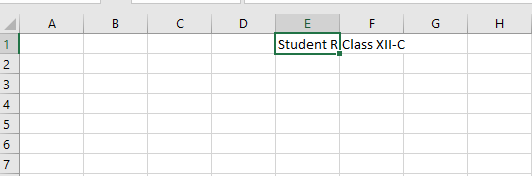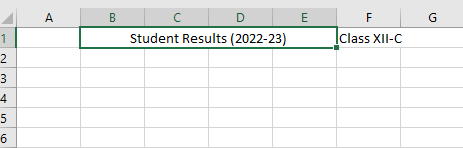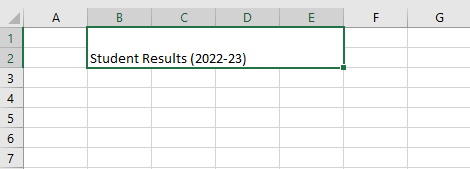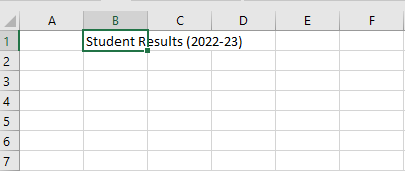GFG App
Open AppBrowser
Continue

# Merge and Unmerge Excel Cells using openpyxl in R

In this article, we are going to learn about Python programs to merge and unmerge excel cells using openpyxl.

### Introduction

Openpyxl is a Python library commonly used for reading and writing Excel Files. In this article, we will be using this library to merge cells in Excel. Merging is helpful when we want to display some special information in order to gain user attention or to just properly display a long word properly. For example, Consider the following sheet. If you want to display a long title. By default, it will appear like this.Here, we can see that before merging, the title appears to be meaningless and less user-friendly. However, if we merge some cells, the heading will appear much more clearly and read-friendly. After merging some adjacent cells, It will look something like this.## Merging cells in excel using Python

Step 1: Installing the required packages, we are using openpyxl to handle tasks such as the creation of a new workbook, updating an existing workbook, querying results, sorting, filtering, etc. Firstly let’s install the package by running the below command in cmd or terminal.

`pip install openpyxl`

Now, we can import the package into our Python program as follows:

```import openpyxl
import os      # for reading the excel file.```

Step 2: Load an existing workbook to work on.

In this step, we are going to select the existing workbook we want to work on. For this, we need the location where the workbook is present in the local system. Let’s store this path in a PATH variable. If the excel file is present in the current working directory, we simply need to get the path of the current directory and join it with the name of our Excel file as follows:

`path=os.path.join(os.getcwd(),"sample.xlsx");`

Now, let’s load the workbook using the library function load_workbook from openpyxl. This function requires the path(from where the workbook has to be loaded) as defined above.

`wb=openpyxl.load_workbook(path)`

Now, let’s open the active worksheet from our workbook wb as follows:

`ws=wb.active`

Or, if we want to open a non-active worksheet. We can open it like this:

`ws=wb["name_of_the_worksheet_here"]`

Here, is the workbook, before merging the cells.Step 3: Now, let’s apply the function merge cells to merge specific cells into one. This function requires the following arguments.

1. start_row: Specifies the starting index of the row to start merging from.
2. start_column: Specifies the starting index of the row to start merging from.
3. end_row: Specifies the last index of the row to end merging.
4. end_column: Specifies the last index of the column to end merging.

Now, let’s use the function to merge rows from 1 to 2 and columns from B to E into a single cell.

`ws.merge_cells(start_row=1, start_column=2, end_row=2, end_column=5)`

After this step, row number 1 to 2 and column number B to E will be merged into a single cell.

Step 4: Saving the workbook to apply the changes.

`wb.save(filename="sample.xlsx")`

Final Code:

## Python3

 `import` `openpyxl ` `import` `os ` `path``=``os.path.join(os.getcwd(),``"sample.xlsx"``) ` `wb``=``openpyxl.load_workbook(path) ` `ws``=``wb.active ` `ws.merge_cells(start_row``=``1``, start_column``=``2``, end_row``=``2``, end_column``=``5``) ` `wb.save(filename``=``"sample.xlsx"``)`

Output:## Unmerging the cells in an excel file using Python

Step 1: Import the required packages.

```import openpyxl
import os      # for reading the excel file.```

Step 2:Open the Excel Working using the load_workbook function from openpyxl.This function accepts a path as a parameter and opens the Excel File.

Here, we are first storing the path of the Excel File in a PATH variable. The name of our Excel File is sample.xlsx and it is presenting the current working directory. So we do something like this:

`path=os.path.join(os.getcwd(),"sample.xlsx")`

Now, let’s load the workbook into our Python code. and open the currently active workbook from it.

```wb=openpyxl.load_workbook(path)
ws=wb.active```

Step 3:Unmerging the cells.

As we can see initially the worksheet looks like this:Here, the cells B1:E2 are merged into a single cell. Let’s unmerge these cells.

To do so, we use the unmerge_cells function from openpyxl which takes the following parameters:

1.start_row: Specifies the starting index of the row to start unmerging from.
2.start_column: Specifies the starting index of the row to start unmerging from.
3.end_row: Specifies the last index of the row to end unmerging.
4.end_column: Specifies the last index of the column to end unmerging.

Now, let’s use the function to unmerge row number 1 to 2 and column number 2 to 5 into individual cells.

`ws.unmerge_cells(start_row=1, start_column=2, end_row=2, end_column=5)`

After this step, the following worksheet will be produced.

Step 4:To save the changes, we can save the worksheet as:

`wb.save(filename="sample.xlsx")`

Final Code:

## Python3

 `import` `openpyxl ` `import` `os ` `path``=``os.path.join(os.getcwd(),``"sample.xlsx"``) ` `wb``=``openpyxl.load_workbook(path) ` `ws``=``wb.active ` `ws.unmerge_cells(start_row``=``1``, start_column``=``2``, end_row``=``2``, end_column``=``5``) ` `wb.save(filename``=``"sample.xlsx"``) `

Output:My Personal Notes arrow_drop_up